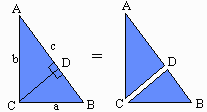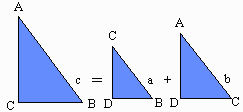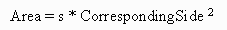## Proof of the Pythagorean Theorem

There are many ways to prove the Pythagorean Theorem. A particularly simple one is to use the scaling relationship for areas of similar figures.Consider any right triangle ABC. Choose point D on the hypotenuse AB, such that line CD is perpendicular to AB. Then the large right triangle ABC is split into two smaller right triangles ADC and BDC.

All three triangles have equal angles and are thereforesimilar, so their areas are related by the scaling formula:for some number "s" that is the same for all three triangles.

(Scroll down to see the rest of the proof.)

For similar shapes, area increases as the square of the lengths of corresponding sides.